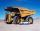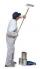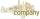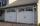Blueberries 2

Lenka and Martin collected eight liters of blueberries in two hours. How long will take to collect blueberries by Lenka itself, if we know that she collect all 2.5 hours less than Martin?

Result

x =  5 h

Solution:Leave us a comment of this math problem and its solution (i.e. if it is still somewhat unclear...):Be the first to comment!To solve this verbal math problem are needed these knowledge from mathematics:

Looking for help with calculating roots of a quadratic equation? Do you have a linear equation or system of equations and looking for its solution? Or do you have quadratic equation?

Next similar math problems:

1. Hectoliters of waterThe pool has a total of 126 hectoliters of water. The first pump draws 2.1 liters of water per second. A second pump pumps 3.5 liters of water per second. How long will it take both pumps to drain four-fifths of the water at the same time?
2. NovakNovak needed to dig up three of the same pit in the garden. The first pit dug father alone for 15 hours. His second dig son helped him and it did that in six hours. The third pit dug son himself. How long it took him?
3. TrucksTruck take you the some amount of clay in 30 hours. Second truck in 40 hours. Trucks carted the clay away some time together then the second truck broke down and the first one completed the job in five hours. What time worked together?
4. WorkThe first worker would need less than 4 hours to complete the task than the other worker. In fact, both workers worked for two hours together, then the first worker did the remaining work himself. In what proportion should the remuneration of the workers b
5. Painters 4One painter will do the job for 12 days. After a 4 1/3 day he comes second to help him and complete work together for 8 days. How long would work the second painter himself?
6. Our houseOur house would paint four painters in 14 days. How long could it paint five painters? How long will it take if two other painters will come to help after 5 days?
7. Digging companiesCompany A would dig a pit for 12 days, company B for 15 days, company C for 20 days and the company D for 24 days. Work began together companies C and D, but after three days joined them other two companies. How long took to dig a pit?
8. RootsDetermine the quadratic equation absolute coefficient q, that the equation has a real double root and the root x calculate: ?
9. Square root 2If the square root of 3m2 +22 and -x = 0, and x=7, what is m?
10. EquationEquation ? has one root x1 = 8. Determine the coefficient b and the second root x2.
11. Holidays - on poolChildren's tickets to the swimming pool stands x € for an adult is € 2 more expensive. There was m children in the swimming pool and adults three times less. How many euros make treasurer for pool entry?
12. Fifth of the numberThe fifth of the number is by 24 less than that number. What is the number?
13. Two masonsTwo masons built the garage together - it took 18 days. If they worked independently, the other would work 15 days more than the first. For how many days would build the garage each mason himhelp?
14. Solve 3Solve quadratic equation: (6n+1) (4n-1) = 3n2
15. DiscriminantDetermine the discriminant of the equation: ?
16. FractionFor what x expression ? equals zero?Find the roots of the quadratic equation: 3x2-4x + (-4) = 0.